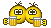# Calculation of Mean anomaly

#### Nikogori

##### Donator
Donator
I'm trying to calculate Mean anomaly which appears in my textbook but I can't get correct answer.

semi major axis a = 26552.305 km
eccentricity e = 0.747411
mean anomaly at epoch M_0 = 24.70°
inclination i = 63.96°
longitude of ascending node Ω = 33.79°
argument of periapsis ω = 243.99°
t – t_0 = 4707393.045 secMean anomaly I get from above equation is M = 711.6°

eccentric anomaly E is shown in the textbook.
E = 157.69°
therefore, required mean anomaly should be M = 141.4°

Can someone tell me where I went wrong? I'm not a student, I have no one to ask math questions...

#### Urwumpe

##### Not funny anymore
Donator
Your formula is correct, if that relieves you a bit. Its mean motion ($\sqrt{\frac{\mu}{a^3}}$) multiplied by time passed since reference ($t-t_0$) shifted by the reference mean anomaly $M_0$
Well, you know how the mean anomaly is defined geometrically? Maybe it helps remember that the mean anomaly maps your real elliptic orbit on a perfect circular orbit that has the same orbit period.

Eccentric anomaly on the other hand is the angle to a virtual point that is created by taking your elliptic orbit and stretching it into a circle.

I also get completely different numbers than you do with the same input numbers, which leads me to the conclusion that $t-t_0$ is not the time of E. I even get different numbers if I "forget" converting the angles into radians.

My results so far:

M from time|351.6°
M from E|157.41°

Last edited:

#### martins

##### Orbiter Founder
Orbiter Founder
I get M = 141.4 deg with the OP's equation (assuming mu is for Earth, which wasn't actually specified).All is well ...

Edit: well, actually M = 3.9381e+04 deg which might matter if you need to count orbits ...

Edit2: As an attempt at a postmortem, I *do* get 711.6 if if forget a set of brackets and only convert the second term to degrees:

Is that what happened?

Edit3: Sorry, the above was assuming that M0 had been converted to radians to start with. So maybe all that happened was that you forgot that the first term gives a result in radians, not degrees.

#### Nikogori

##### Donator
Donator
Your formula is correct, if that relieves you a bit. Its mean motion ($\sqrt{\frac{\mu}{a^3}}$) multiplied by time passed since reference ($t-t_0$) shifted by the reference mean anomaly $M_0$

Thank you. Actually my textbook says $\sqrt{\frac{a^3}{\mu}}$. It took me a day to realize it was a typo.

So maybe all that happened was that you forgot that the first term gives a result in radians, not degrees.

Thank you! That was what actually happened to me. Now I got the correct answer.

#### martins

##### Orbiter Founder
Orbiter Founder
Using degrees as angular units sometimes makes for easier human reading, but is useless for any trigonometric calculations. To avoid confusion, I'd recommend to

- convert all input angular data to radians (or angular velocities to radians/s, etc.)